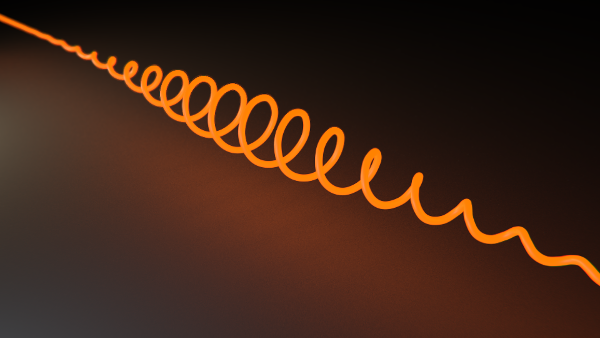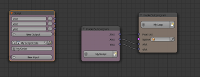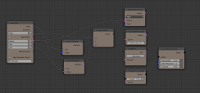## Animation Node experiment - script node spiral

For this animation I used the python script node of the Animation-Node-Addon to create a list of coordinates for a bezier-curve.First script node and connected it to a loopI used the following script to return 3 float lists containing the x, y and z coordinates of the control points.

``````import math
lenght = 100
zlist = [ x/4 for x in range(-lenght,lenght)]

ylist = [math.sin(x) * math.exp( - x**2/1000) for x in range(-lenght,lenght)]
xlist = [math.cos(x)  * math.exp( - x**2/1000) for x in range(-lenght,lenght)]
``````

Then I used a loop to add the points to the curve using this node setup. I´m pretty sure this is not the most elegant way to solve the problem, but it does the jobThe I duplicated the resulting curve and used a emission shader on the first one and a slightly transparent diffuse shader on the second curve. The animation is created using a build modifier on each node.